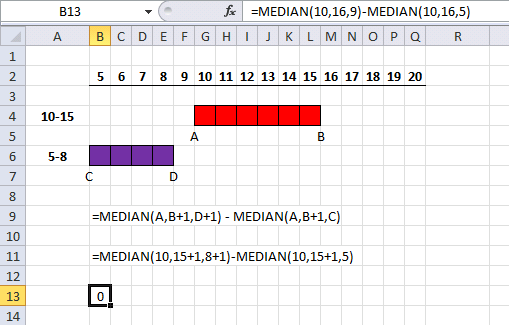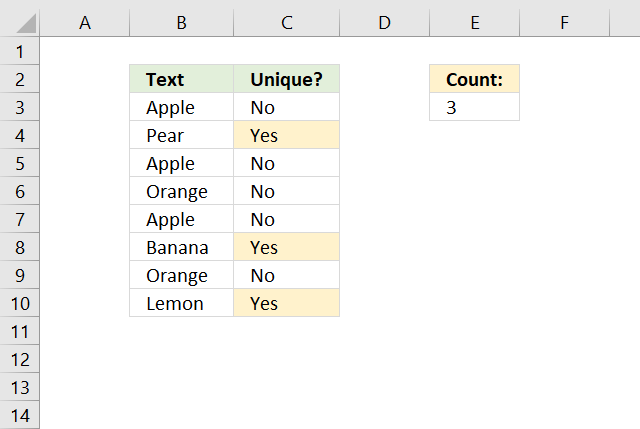How do I compare two different lists?

The following articles demonstrate how to extract values that either is shared by both columns or not:

How do I compare two different data sets?

A dataset is a group of related values, often each row contains values that form a record. Data organized vertically is not that common.

How can I extract values shared/not shared between two cell ranges?

The cell range in the articles below contains values that are NOT organized by row, in other words, they are not related.

How can I automatically plot date ranges in a schedule?

This article Shift Schedule explains how to show date ranges in a schedule using formulas. You can also do the opposite, Get date ranges from a schedule using a formula.

The following article demonstrates a calendar that highlights events: Yet another excel calendar or: Highlight events in a calendar I recommend that you check out the Calendar category.

How can I find overlapping date ranges?

The easiest way to check if a date range is overlapping another date range is shown in the following article: Identify overlapping date ranges

This article shows how to highlight overlapping date ranges based on a condition: Highlight records based on overlapping date ranges and a conditionThis article explains how to calculate overlapping days between two date ranges: Days contained in a range that overlap another range

To count overlapping days across multiple date ranges, see these articles:
Count overlapping days
Count overlap. days(1)
Count overlap. days(2)

Check out the overlapping category for more articles.

How can I identify overlapping time ranges?

Use MEDIAN function to calculate overlapping ranges

How can I automatically create date ranges?

Follow the instructions on this web page: Create a date range [Formula]

How do I combine or merge two columns?

Merge two columns

How do I combine or merge three columns?

Merge three columns into one list

How do I combine or merge two tables?

Merge tables based on a condition

How do I count a pattern in a cell value?

Count a given pattern in a cell value

How do I count unique distinct values?

Count unique distinct values

Count unique distinct values based on a condition

Count unique distinct values that meet multiple criteria

How do I count unique values?A unique value is a value that exists only once in a list, for example, value Pear exists only once in the list above so that value is counted as a unique value.

There are three unique values in the list above: Pear, Banana and Lemon. All other values has at least one duplicate value.

Formula in cell E3:

=SUMPRODUCT((COUNTIF(\$B\$3:\$B\$10,\$B\$3:\$B\$10)=1)*1)

Explaining formula in cell E3

Step 1 - Count each value

The COUNTIF function counts the number of cells that equals a given value, however, if we use multiple values the function returns an array of values containing the number of values for each given value.

COUNTIF(\$B\$3:\$B\$10,\$B\$3:\$B\$10)

becomes

COUNTIF({"Apple"; "Pear"; "Apple"; "Orange"; "Apple"; "Banana"; "Orange"; "Lemon"},{"Apple"; "Pear"; "Apple"; "Orange"; "Apple"; "Banana"; "Orange"; "Lemon"})

and returns {3; 1; 3; 2; 3; 1; 2; 1}.

Step 2 - Identify unique values

We know that a value is a unique value if the corresponding number in the array is equal to 1. The equal sign allows us to compare each number in the array. It returns the boolean value TRUE or FALSE.

COUNTIF(\$B\$3:\$B\$10, \$B\$3:\$B\$10)=1

becomes

{3; 1; 3; 2; 3; 1; 2; 1}=1

and returns {FALSE; TRUE; FALSE; FALSE; FALSE; TRUE; FALSE; TRUE}.

Step 3 - Multiply with 1

The SUMPRODUCT function can't add boolean values so we must convert the boolean values to their numerical equivalents 1 and 0 (zero).

The parentheses let you change the order of calculation, we want to multiply the array with 1 and nothing else.

(COUNTIF(\$B\$3:\$B\$10,\$B\$3:\$B\$10)=1)*1

becomes

({FALSE; TRUE; FALSE; FALSE; FALSE; TRUE; FALSE; TRUE})*1

and returns {0;1;0;0;0;1;0;1}.

SUMPRODUCT((COUNTIF(\$B\$3:\$B\$10,\$B\$3:\$B\$10)=1)*1)

becomes

SUMPRODUCT({0;1;0;0;0;1;0;1})

and returns 3.

How do I count repetead contiguous values

Count contiguous values

How do I extract duplicate values?